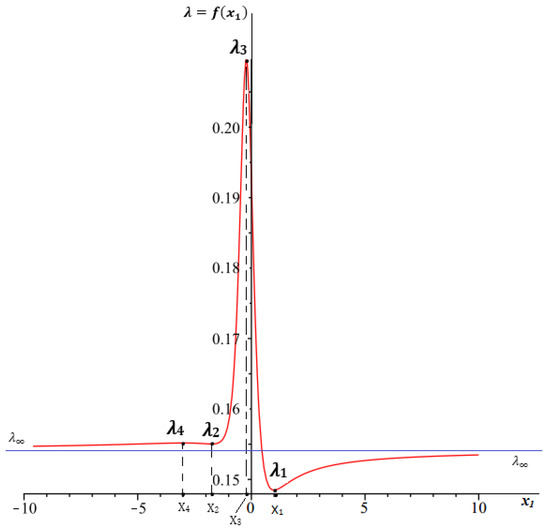Next Article in Journal
Hydromagnetic Flow of Micropolar Nanofluid
Previous Article in Journal
Core Stability and Symmetry of Youth Female Volleyball Players: A Pilot Study on Anthropometric and Physiological Correlates
Previous Article in Special Issue
Modifications of Gravity Via Differential Transformations of Field Variables
Open AccessArticle

# Exponential Cosmological Solutions with Three Different Hubble-Like Parameters in (1 + 3 + k1 + k2)-Dimensional EGB Model with a Λ-Term

byK. K. Ernazarov 1 andV. D. Ivashchuk 1,2,*1
Institute of Gravitation and Cosmology, Peoples’ Friendship University of Russia (RUDN University), 6 Miklukho-Maklaya Street, 117198 Moscow, Russia
2
Center for Gravitation and Fundamental Metrology, VNIIMS, 46 Ozyornaya Street, 119361 Moscow, Russia
*
Author to whom correspondence should be addressed.
Symmetry 2020, 12(2), 250; https://doi.org/10.3390/sym12020250
Received: 15 January 2020 / Revised: 31 January 2020 / Accepted: 2 February 2020 / Published: 6 February 2020
A D-dimensional Einstein–Gauss–Bonnet model with a cosmological term $Λ$ , governed by two non-zero constants: $α 1$ and $α 2$ , is considered. By restricting the metrics to diagonal ones, we study a class of solutions with the exponential time dependence of three scale factors, governed by three non-coinciding Hubble-like parameters: $H > 0$ , $h 1$ , and $h 2$ , obeying $3 H + k 1 h 1 + k 2 h 2 ≠ 0$ and corresponding to factor spaces of dimensions: 3, $k 1 > 1$ , and $k 2 > 1$ , respectively, with $D = 4 + k 1 + k 2$ . The internal flat factor spaces of dimensions $k 1$ and $k 2$ have non-trivial symmetry groups, which depend on the number of compactified dimensions. Two cases: (i) $3 < k 1 < k 2$ and (ii) $1 < k 1 = k 2 = k$ , $k ≠ 3$ , are analyzed. It is shown that in both cases, the solutions exist if $α = α 2 / α 1 > 0$ and $α Λ > 0$ obey certain restrictions, e.g., upper and lower bounds. In Case (ii), explicit relations for exact solutions are found. In both cases, the subclasses of stable and non-stable solutions are singled out. Case (i) contains a subclass of solutions describing an exponential expansion of $3 d$ subspace with Hubble parameter $H > 0$ and zero variation of the effective gravitational constant G. View Full-Text
Show FiguresFigure 1

MDPI and ACS Style

Ernazarov, K.K.; Ivashchuk, V.D. Exponential Cosmological Solutions with Three Different Hubble-Like Parameters in (1 + 3 + k1 + k2)-Dimensional EGB Model with a Λ-Term. Symmetry 2020, 12, 250.